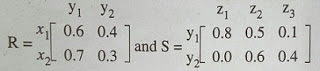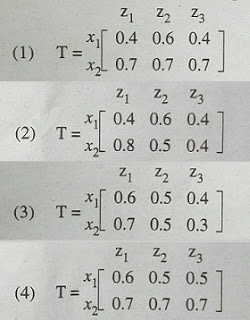## Discussion Forum

 Que. Let R and S be two fuzzy relations defined as follows. Then, the resulting relation, T, which relates elements of universe x to the elements of universe z using max-min composition is given by:a. (1) b. (2) c. (3) d. (4) Answer:(3)